Next: Untying loops Up: 7: GEOMETRICAL PROBLEMS OF Previous: 7: GEOMETRICAL PROBLEMS OF

## Interference analysis

If we have two waves with different apparent velocities that interfere with each other, then it is reasonable to apply wave-field continuation into the homogeneous model because these waves can be separated as a result of propagation in a continued field. The consideration is very simple. If both waves are observed in the interval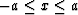and the k-th one has travel time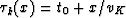(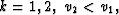see Figurea), then as the result of forward continuation into the medium with velocity, vc<min{v1,v2,} we obtain waves with eiconals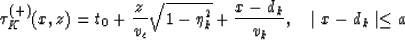where,see Figureb. It is easy to check that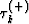satisfies to the eiconal equation and to the boundary condition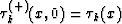.

The choice of velocity vc does not depend on the velocity in the true model of the medium, but only on the condition of wave resolution. We have time resolution if(where T0 is the duration of the pulse) and spatial resolution if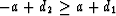. It is very easy to derive proper conditions in terms of z, a,v1, v2 and Vc. For example, we have spatial resolution ifwhere. But we shall not develop these considerations since there are more powerful solution. Let us return to the condition Vc <min{V1, V2}. Does it have a sense to refuse from the condition?

Let one of the waves be nondesirable (for example, one which has less value of apparent velocity: v2 < v1 ). Then the best choice of the velocity for continuation is v=vc:v2 <vc<v1. It is well known that apparent velocity is always more than the velocity in the medium. When v=vc, then the wave with velocity v2<vc will not be continued into a medium at all! To be exact, it will propagate in the model as an evanescent wave (along the surface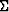)whose amplitude very quickly (exponentially) diminishes with increasing |z|.

In fact, the operators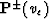act as fan filters for the fan of velocities

|v|>vc.

In order to improve the quality of resolution, we can introduce preliminary time shifts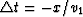.Afterward waves have new apparent velocities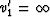and v'2 =vn. Now we may apply the operatorat vc<vn. The big advantage of this approach is that it is not necessary that apparent velocities v1 and v2 (or v'1 and v'2) not be constant along a profile. The only essential condition is v1(x)<vc<v2(x).

The Figureillustrates interference analysis for the situation when V1 changes in the interval (5 km/s, 6.3 km/s) and V2 changes in the interval (3 km/s, 4.1 km/s). Figurea shows the original record, Figureb shows the result of standard fan filtration in the velocity fan (4.6 km/s, 7.1 km/s), and Figurec shows the result of the forward continuation with Vc = 4.9 km/s.Next: Untying loops Up: 7: GEOMETRICAL PROBLEMS OF Previous: 7: GEOMETRICAL PROBLEMS OF
Stanford Exploration Project
1/13/1998